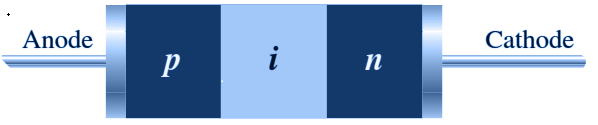# PIN Diode

The Diodes having P-N junction are the popular types of diode used so far in various applications. One of those types of circuits is PIN diode. This diode is used in a wide range of areas. It is very good in the applications of RF switching and the structure is useful in the photodiodes.

## What is PIN Diode?

A PIN diode can be defined as:

A diode with a wide and undoped intrinsic semiconductor region between a p-type and an n-type semiconductor region.

It has an undoped intrinsic semiconductor region between p-type and an n-type semiconductor.

• The PIN diode was first used in the year 1952 as a low frequency and high power rectifier. It was even used for microwave applications and as a photo-detector as it is said to be a good absorber of light.
• A PIN diode is a type of diode having undoped intrinsic semiconductor region placed between two regions called p-type semiconductor and the n-type semiconductor region. These two regions are primely heavily doped as they find applications for ohmic contacts.

## Characteristics of Pin Diode

Some of the PIN Diode characteristics are given in the points below.

• The capacitance of PIN diode is independent of bias level as the net charge is said to be very less in the intrinsic layer
• PIN diode possesses very low reverse recovery time
• The Diode obeys standard diode equation for all the low-frequency signals.
• This diode appears like a resistor than any other non- linear devices and is said to produce no distortion or rectification.

### Structure and Working of a Pin Diode

The PIN diode comprises a semiconductor diode having three layers naming P-type layer, Intrinsic layer and N-type layer as shown in the figure below. The P and N regions are there and the region between them consists of the intrinsic material and the doping level is said to be very low in this region.  The thickness of the intrinsic layer is very narrow, which ranges from 10 – 200 microns. The P region and the N-type regions are known to be heavily doped.Structure of a Pin Diode

The changes in the properties of the diode are known from the intrinsic material. These diodes are made of silicon. The intrinsic region of the PIN diode acts like an inferior rectifier which is used in various devices such as attenuators, photodetectors, fast switches, high voltage power circuits, etc.

You may also want to check out these topics given below!

## Applications of PIN Diode

PIN Diode has a wide range of applications coming in various fields of physics like radio frequency switch, microwave switch, radiofrequency attenuator, microwave attenuator, photodetector, etc. Some applications are given below:

• These diodes are used in the RF and also for microwave switches and microwave variable attenuators since they are said to have low capacitance.
• They are used in Photodetectors and photovoltaic cell and the PIN photodiodes are used for fibre optic network cards and also switches.
• These diodes are effectively used for RF protection circuits and it can also be utilized as an RF switch.
• The PIN photodiode is also used to detect X-rays and gamma rays photons.

### PIN Diode Questions (FAQs)

Q1. Name the material that is used in the PIN diode.

Ans: Silicon is used because it has power handling capacity.

Q2. How many layers of semiconductors are there in PIN diode?

Ans: There are three layers of semiconductors and they are p-type and n-type which are narrow and an intrinsic layer which is a thick region.

Q3. What happens to the resistance of the PIN diode with a positive bias voltage?

Ans: It decreases.

Q4. What is the forward resistance of the PIN diode?

Ans: $$R_{F}=\frac{W}{\sigma N}$$

The forward resistance depends on the width, current density and positive carrier concentration of the diode.

Q5. What is the value of forward current in frequency models?

Ans: IF=A(μPP+μNN)q

Where,

• μP is the mobility of p-type charge carriers
• μN is the mobility of n-type charge carriers.

Q6. What happens in the PIN diode for low-frequency model?

Ans: For low-frequency model, there is a decrease in resistance and increase in reactance.

Q7. When does the PIN diode act as a variable capacitor?

Ans: It acts as a variable capacitor when it is reverse biased.

Q8. When does the PIN diode act as a variable resistor?

Ans: It acts as a variable resistor when it is forward biased.

Stay tuned with BYJU’S to learn more Physics concepts with the help of interactive video lessons.

Test your Knowledge on Pin Diode